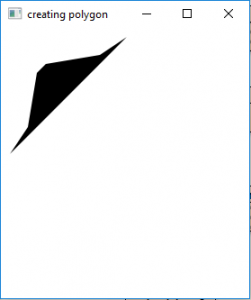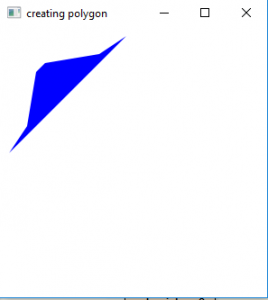Related Articles
JavaFX | Polygon with examples
• Last Updated : 25 Oct, 2019

Polygon is a part of the JavaFX library. Polygon class creates a polygon with the given set of x and y coordinates. Polygon class inherits the shape class.

Constructors of the class are:

1. Polygon(): creates a empty polygon with no set of defined coordinates of points(vertices)
2. Polygon(double points[])creates a polygon with a set of defined coordinates of points(vertices)

Commonly used methods:

method explanation
getPoints() Gets the coordinates of the Polygon vertices.
setFill(Paint p) sets the fill for the polygon

Below programs will illustrate the Polygon class of JavaFX:

1. Program to create a polygon with a given set of vertices: This program creates a Polygon indicated by the name polygon. The coordinates for the vertices of the polygon are passed as arguments. The Polygon will be created inside a scene, which in turn will be hosted inside a stage. The function setTitle() is used to provide title to the stage. Then a Group is created, and the polygon is attached. The group is attached to the scene. Finally, the show() method is called to display the final results.

 `// Java Program to create a polygon with a given set of vertices ` `import` `javafx.application.Application; ` `import` `javafx.scene.Scene; ` `import` `javafx.scene.control.Button; ` `import` `javafx.scene.layout.*; ` `import` `javafx.scene.paint.Color; ` `import` `javafx.scene.shape.Polygon; ` `import` `javafx.scene.control.*; ` `import` `javafx.stage.Stage; ` ` `  `import` `javafx.scene.Group; ` `public` `class` `polygon_0 ``extends` `Application { ` ` `  `    ``// launch the application ` `    ``public` `void` `start(Stage stage) ` `    ``{ ` `        ``// set title for the stage ` `        ``stage.setTitle(``"creating polygon"``); ` ` `  `        ``// coordinates of the points of polygon ` `        ``double` `points[] = { ``10``.0d, ``140``.0d, ``30``.0d, ``110``.0d, ``40``.0d, ` `          ``50``.0d, ``50``.0d, ``40``.0d, ``110``.0d, ``30``.0d, ``140``.0d, ``10``.0d }; ` ` `  `        ``// create a polygon ` `        ``Polygon polygon = ``new` `Polygon(points); ` ` `  `        ``// create a Group ` `        ``Group group = ``new` `Group(polygon); ` ` `  `        ``// create a scene ` `        ``Scene scene = ``new` `Scene(group, ``500``, ``300``); ` ` `  `        ``// set the scene ` `        ``stage.setScene(scene); ` ` `  `        ``stage.show(); ` `    ``} ` ` `  `    ``public` `static` `void` `main(String args[]) ` `    ``{ ` `        ``// launch the application ` `        ``launch(args); ` `    ``} ` `} `

Output:2. Program to create a polygon with a given set of vertices and specified fill: This program creates a Polygon indicated by the name polygon. The coordinates for the vertices of the polygon are passed as arguments. The function set Fill() is used to set the fill of the polygon. The Polygon will be created inside a scene, which in turn will be hosted inside a stage. The function setTitle() is used to provide title to the stage. Then a Group is created, and the polygon is attached. The group is attached to the scene. Finally, the show() method is called to display the final results.

 `// Java Program to create a polygon with a ` `// given set of vertices and specified fill ` `import` `javafx.application.Application; ` `import` `javafx.scene.Scene; ` `import` `javafx.scene.control.Button; ` `import` `javafx.scene.layout.*; ` `import` `javafx.scene.paint.Color; ` `import` `javafx.scene.shape.Polygon; ` `import` `javafx.scene.control.*; ` `import` `javafx.stage.Stage; ` ` `  `import` `javafx.scene.Group; ` `public` `class` `polygon_1 ``extends` `Application { ` ` `  `    ``// launch the application ` `    ``public` `void` `start(Stage stage) ` `    ``{ ` `        ``// set title for the stage ` `        ``stage.setTitle(``"creating polygon"``); ` ` `  `        ``// coordinates of the points of polygon ` `        ``double` `points[] = { ``10``.0d, ``140``.0d, ``30``.0d, ``110``.0d, ``40``.0d, ` `            ``50``.0d, ``50``.0d, ``40``.0d, ``110``.0d, ``30``.0d, ``140``.0d, ``10``.0d }; ` ` `  `        ``// create a polygon ` `        ``Polygon polygon = ``new` `Polygon(points); ` ` `  `        ``// set fill for the polygon ` `        ``polygon.setFill(Color.BLUE); ` ` `  `        ``// create a Group ` `        ``Group group = ``new` `Group(polygon); ` ` `  `        ``// create a scene ` `        ``Scene scene = ``new` `Scene(group, ``500``, ``300``); ` ` `  `        ``// set the scene ` `        ``stage.setScene(scene); ` ` `  `        ``stage.show(); ` `    ``} ` ` `  `    ``public` `static` `void` `main(String args[]) ` `    ``{ ` `        ``// launch the application ` `        ``launch(args); ` `    ``} ` `} `

Output:3. Note: The above programs might not run in an online IDE please use an offline IDE.

Attention reader! Don’t stop learning now. Get hold of all the important Java Foundation and Collections concepts with the Fundamentals of Java and Java Collections Course at a student-friendly price and become industry ready.

My Personal Notes arrow_drop_up
Recommended Articles
Page :